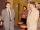# Four numbers

The first number is 50% second, the second number is 40% third, the third number is 20% of the fourth. The sum is 396. What are the numbers?

Result

a =  12
b =  24
c =  60
d =  300

#### Solution:Leave us a comment of example and its solution (i.e. if it is still somewhat unclear...):

Showing 0 comments:Be the first to comment!#### To solve this example are needed these knowledge from mathematics:

Our percentage calculator will help you quickly calculate various typical tasks with percentages. Do you have a linear equation or system of equations and looking for its solution? Or do you have quadratic equation?

## Next similar examples:

1. The largerThe larger of two numbers is nine more than four times the smaller number. The sum of the two numbers is fifty-nine. Find the two numbers.
2. SchoolsThree schools are attended by 678 pupils. To the first attend 21 students more and to the third 108 fewer students than to second school. How many students attend the schools?
3. Two workersTwo workers make 138 parts/items. The first one produces 30% more than the second. How many items will each produce?
4. Perctentages35% of what number is 35?
5. PercentCalculate how many % is the number 26.25 less than the number 105.
6. PercentsHow many percents is 900 greater than the number 750?
7. PupilsThere are 350 girls in the school, and the other 30% of the total number of pupils are boys. How many pupils does the school have?
8. Football match 4In a football match with the Italy lost 3 goals with Germans. Totally fell 5 goals in the match. Determine the number of goals of Italy and Germany.
9. PersonsPersons surveyed:100 with result: Volleyball=15% Baseball=9% Sepak Takraw=8% Pingpong=8% Basketball=60% Find the average how many like Basketball and Volleyball. Please show your solution.
10. Cinema ticketsCinema sold 180 tickets this Thursday, which is 20%. Monday 14%, Tuesday 6%, Wednesday 9%, Friday 24%, Saturday 12%, and Sunday 15%. How many tickets were sold per week?
11. Test pointsIf you earned 80% of the possible 40 points, how many points did you miss to get 100%?
12. Profit gainIf 5% more is gained by selling an article for Rs. 350 than by selling it for Rs. 340, the cost of the article is:
13. Three friendsThe three friends spent 600 KC in a teahouse. Thomas paid twice as much as Paul. Paul a half less than Zdeněk. How many each paid?
14. EquationsSolve following system of equations: 6(x+7)+4(y-5)=12 2(x+y)-3(-2x+4y)=-44
15. Rabbits 3Viju has 40 chickens and rabbits. If in all there are 90 legs. How many rabbits are there with Viju?
16. TheatroTheatrical performance was attended by 480 spectators. Women were in the audience 40 more than men and children 60 less than half of adult spectators. How many men, women and children attended a theater performance?
17. Family parcelsIn father will he divided the land so that the older son had three bigger part than younger son. Later elder son gave 2.5 ha field to younger and they had both the same. Determine the area of family parcel.